# Linear Algebra and Structural Engineering

Linear Algebra is used quite heavily in Structural Engineering. This is for a very simple reason. The analysis of a structure in equilibrium involves writing down many equations in many unknowns. Often these equations are linear, even when material deformation (i.e. bending) is considered. This is exactly the sort of situation for which linear algebra is the best technique. Consider, for example, the following two dimensional truss: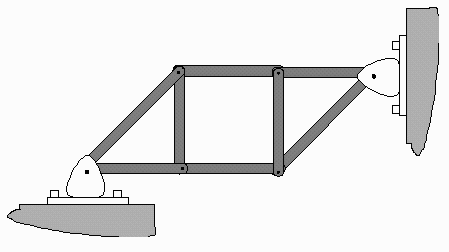The beams are joined together by smooth pins, and the supports are fastened so that they cannot be moved. A simple analysis, using the method of joints, assumes that external forces will only act at the joints, and that the beams are perfectly rigid. This allows for only longitudinal forces in the beams. Given these assumptions, the truss is stable if and only if the vertical and horizontal components of the forces at each joint sum to zero.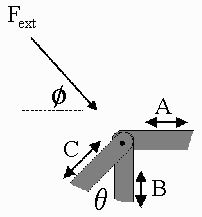For example, in the joint shown to the left, the beams are under compression. Their internal forces, therefore, tend to act against the compression and out against the joint. Static equilibrium demands that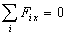and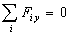. In particular, using the capital letters to denote the magnitude of force along a beam,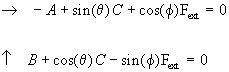Because each joint will give such equations, the total number of equations that can be written, under our assumptions, is twice the number of joints. Normally, we are interested in finding the internal beam forces and the reaction forces at the support under some external load. Thus the number of unknowns is the number of beam forces plus the number of reaction forces.

Here are some examples where we can say quite a bit about the truss only by counting equations and looking at the basic geometry: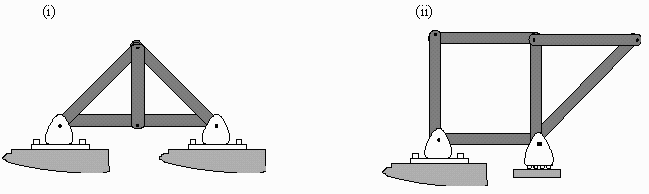The truss in case (i) is stable for certain. This is because the basic buliding blocks are triangular shapes. The triangle is a stable geometry in the sense that there exists only one unique triangle with the same dimensions (the Side-Side-Side Theorem). Thus, under our assumption of perfect rigidity, the truss cannot move even under some external load. There are 4 joints, and therefore 8 equations of static equilibrium. However, there are 5 beams and 4 reaction forces (normal forces fixing the supports), for a total of 9 unknowns. We there have too few equations to determine a unique solution. The truss is said to be indeterminate. To actually solve for the forces in the beams, the material properties of the beams have to be considered. This involves the stiffness of the beams as well as the possible existence of bending moments. Analysis of this truss is thus beyond our simple static equilibrium analysis.

The truss in case (ii) is a different story. Since it is not constructed solely from triangles, there is no guarantee that it is geometrically stable. Moreover, there are 10 equations (from 5 joints), 6 beams and at most 3 reaction forces (the roller can only applies a normal force in the up direction). Thus even if the net external force is in the down direction, the system of equations consists of 10 equations with 9 unknowns. This system is over-determined and, in general, will not yield a solution. This is because there are not enough ‘variables’ to compensate and resist every conceivable configuration of forces.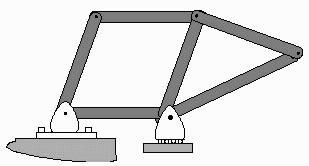For example, a little horizontal push on the leftmost beam will result in the collapse of the truss (see diagram at right).

## Is the First Truss Stable?

Let’s get back to the first truss and analyse it. It is quickly apparent that it has the right number of equations to match the number of unknowns. Because it is not made up solely of triangles, the stability of the truss is not a clear matter. To analyse, we subject the truss to some hypothetical load, write down the static equilibrium set of equations, and try to solve.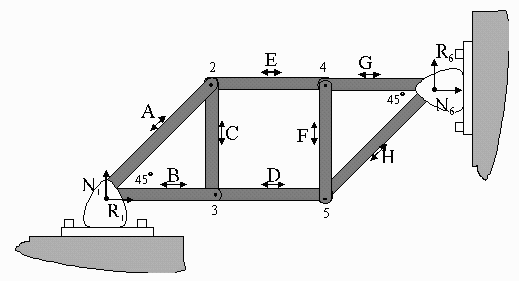Assuming compressive forces, as shown, and some kind of arbitrary loads at each joint, this is the set of equations that results: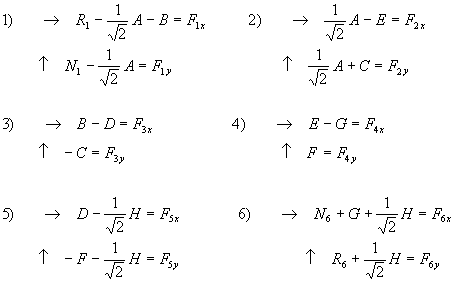The above system of equations can be written in matrix form as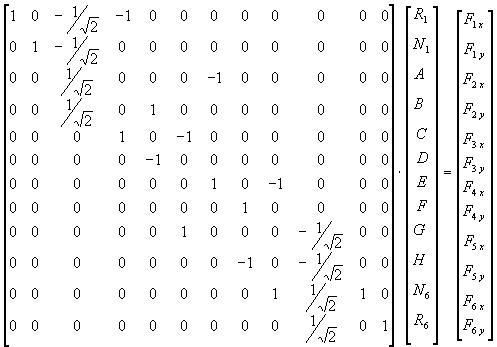This can be solved for any arbitrary external force configuration if and only if we can invert the matrix, A, on the left hand side. Notice that this matrix depends only on the geometry of the truss and not on the load. The matrix can be inverted using Mathematica, Maple, Excel or even a hand held calculator such as the HP 48G. Here is the inverse: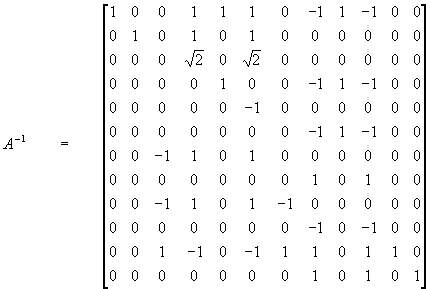The fact that this matrix exists assures us that the truss is stable.Written by Jacob Sagi, October 7, 1997